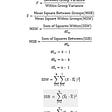# Hypothesis Testing for Dummies

Hypothesis Testing is one of the essential topics to get a better and solid understanding of the derived result. Also, for me, it was one of those topics that baffled my mind for days and left me confused. The reason being it is not just technical but also very intuitive, so I plan to share my findings and help others.

The whole tug of war is about deciding which hypothesis to accept and which to reject among null hypothesis and alternate hypothesis. This will be determined totally on the experiment we perform along with the accepted significance level. Now, this might look a bit complicated and full of jargon like a null hypothesis or alternate hypothesis or significance level; but be with me we will make it through this rough journey quite smoothly.

Steps for Hypothesis Testing

1. Figuring out the null hypothesis from the problem statement

2. State the null hypothesis

3. Choose what kind of test to perform

4. Either support or reject the null hypothesis based on the test result

Hypothesis

A hypothesis is an educated guess about something in the world around you. It should be testable, either by experiment or by observation.

Example: We all know that UV rays are quite harmful to the eyes so one can give a hypothesis that UV light is the cause for blindness.

Hypothesis Statement

If we are going to propose a hypothesis, it’s customary to write a statement. The statement will look like this:

“If I (Do this to an independent variable) then (this will happen to the dependent variable).”

For example, if I decrease the amount of water given to herbs, the herbs will increase.

Hypothesis Testing

Hypothesis testing in statistics is a way for us to test the results of a survey or experiments to see if we have meaningful results. The objective is to test whether the arrived outcome has happened by chance or it is a genuine case. This can be done by figuring out the odds and comparing it with the significance level. If the result seems biased or falls below the significance level then the experiment is non-repeatable and has little use.

Null Hypothesis

The null hypothesis is always the accepted fact. The fact needs to be carefully identified by reading the problem statement.

Let us consider an example, if knee surgery patients go to physical therapy twice a week, their surgery will be longer. The average recovery time for knee surgery patients is 8.2 weeks.

Here we will look into our hypothesis statement and find our null hypothesis i.e., we are looking for that facts or ideas that are nullifiable.

Thus, our null hypothesis will be “The average recovery time is 8.2 weeks”. Now, why do we choose this as our null hypothesis? Because this is a stated fact in our word problem and also can be nullifiable.

H0: The average recovery time is 8.2 weeks

Alternate Hypothesis

An alternate Hypothesis is nothing but just the opposite or opposing statement to that of our null hypothesis.

Thus, for our above example, an alternate hypothesis is

H1: The average recovery time more than 8.2 weeks.

How to reject or accept the Null Hypothesis?

Deciding on what to do with the null hypothesis isn’t always as easy as it’s the stated fact. Therefore, we reject the null hypothesis if and only if the statistic falls in the critical region.

Now, what is this critical region? This is also called a rejection region such that if any test statistic falls in that region, we accept the alternate hypothesis, and this region is decided by significance level.

Now, what is a significance level? The significance level, also known as alpha or α, is a measure of the strength of the evidence that must be present in your sample before you will reject the null hypothesis and conclude that the effect is statistically significant. The researcher determines the significance level before experimenting.

The significance level is the probability of rejecting the null hypothesis when it is true. For example, a significance level of 0.05 indicates a 5% risk of concluding that a difference exists when there is no actual difference. Lower significance levels mean that you require more substantial evidence before you will reject the null hypothesis.

Commonly we take 0.05 as our significance level by default, but it depends on your task to choose the significance level.

Let’s understand all the above with an example:

Problem Statement: Given a coin, determine if the coin is biased towards heads or not.

1. Design an experiment: Let us flip a coin five times and count the number of heads. Here count of heads will be our test statistic.

2. Perform experiment: let’s flip our coin five times

3. After experimenting, we found out that we got heads five heads, so let’s formalize if P(X=5|coin is not biased towards heads), here our test statistic is ‘X’ such that X = count of heads

4. Deciding null hypothesis: Coin is not biased towards heads

5. Determining a significance level: For our example, we will take a default value that is 0.05

6. Now let’s talk about the problem a bit in-depth before going into the step of accepting or rejecting the null hypothesis. If we toss our coin five times, then our sample space will be 5 * 5 = 25 out of this 25 times; if the coin is not biased towards heads, then the chances of getting five heads is 1/25, which is equal to 0.04. Here 0.04 is our p-value or probability of 4%, now let’s move to our final step.

7. Deciding whether to accept or reject the null hypothesis: From the above in-depth analysis, we found that the probability of finding five heads is 4%, and also, we have set our significance level as 0.05 that is 5% hence our test statistics of the number of 5 heads in a row given our coin is not biased towards heads is less than our significance value if wee formalize it, it will be something like P (X=5|coin is not biased towards heads) < significance value

8. Result: We reject our null hypothesis and accept our alternate hypothesis.

References:

2. Significance level by Jim Frost [link]

--

--

--

## More from Analytics Vidhya

Analytics Vidhya is a community of Analytics and Data Science professionals. We are building the next-gen data science ecosystem https://www.analyticsvidhya.com

## Expectation & Variance of OLS Estimates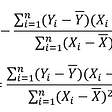## Towards a simple theorem prover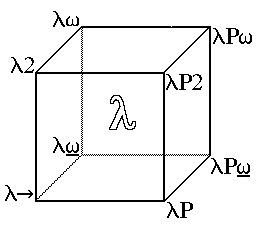## Scientific Computation using Matlab: part2## Bayes Rule: Intuitive and Mathematical understanding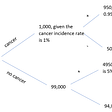## A Little Bit of Math to Reduce False Positives of Signals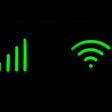## Mathematical Economics: The Good and The Bad## The Factorization of the Ninth Fermat Number## Ujjawalagrawal

I love automation that is AI helps me be more lazy hence I thrive for more knowledge towards AI to attain maximum laziness.

## PCA — (Principal Component Analysis) The best way to look at data smartly## Mixed Methods Data Science: Qualitative Sensibilities